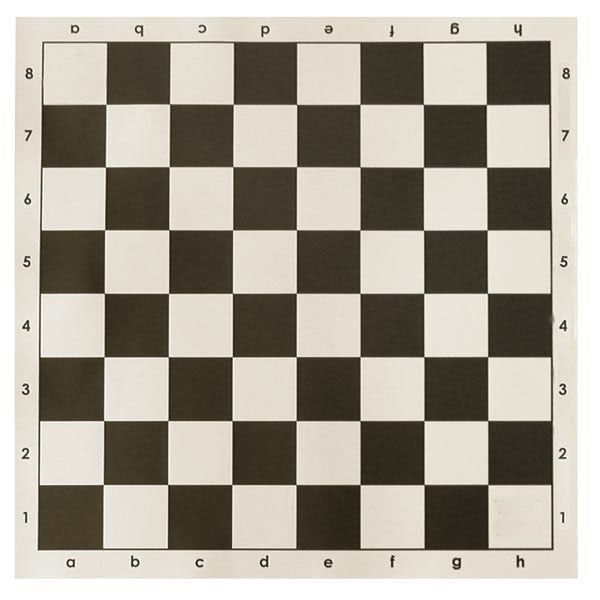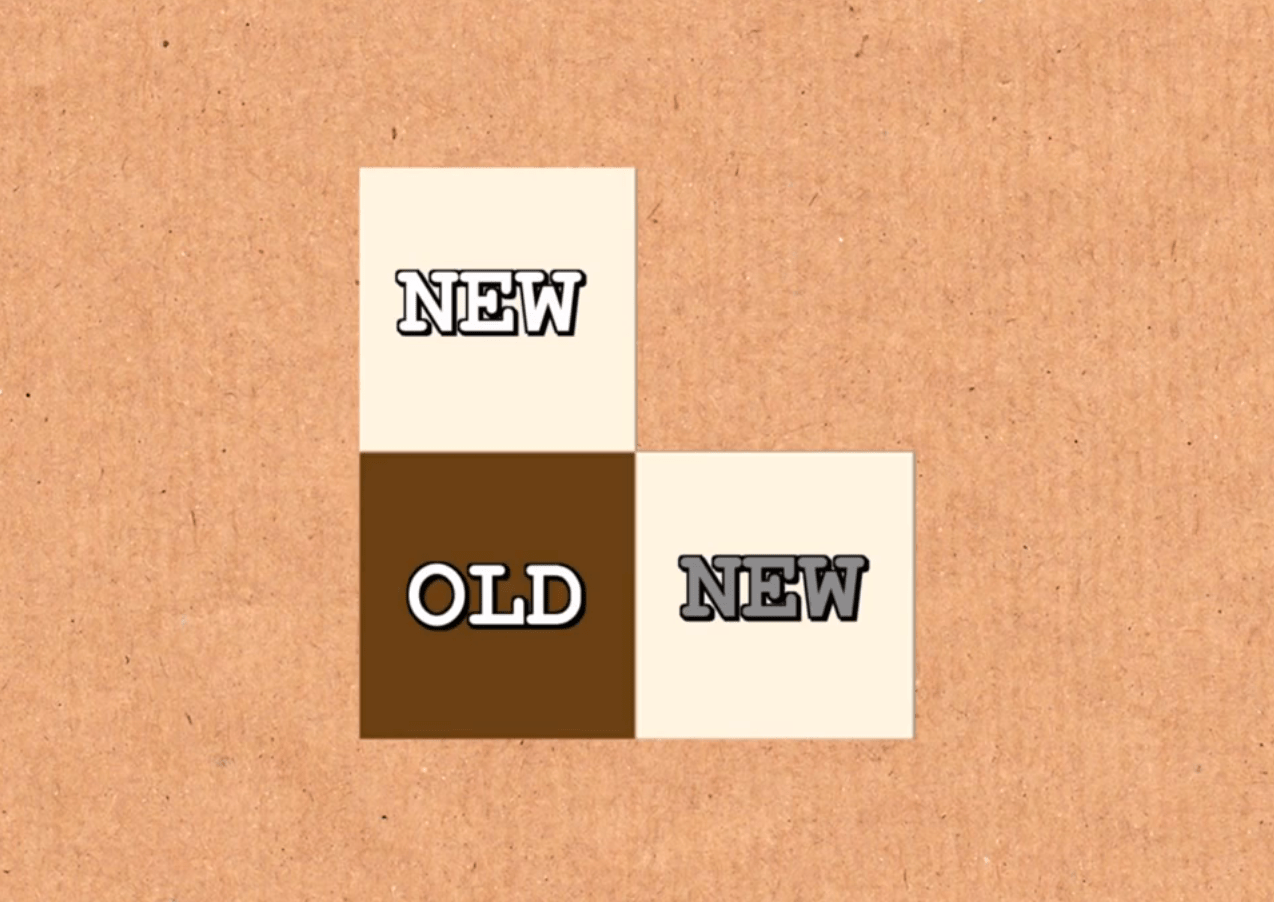# 棋盘上的越狱游戏

Youtube上面有一个很有趣的玩弄数字的频道Numberphile，最近它们发布了一个视频来解释一个很古旧的谜题的解法。这个谜题的规则很简单，问题看上去也很简单，可是当你去尝试解决的时候却会发现这货其实并没有那么简单，最有趣的是，这个最后看上去好像很复杂的问题其实只需要会四则运算就能解决，听上去是不是很好玩呢？如果你的英文还行，访问Youtube也没有障碍的话，现在就可以点击上面的链接去一探究竟。当然如果你懒，也可以听我在这里说，毕竟这就是这篇文章的目的。.

.

.

.

.

.

.

.

.

.$$S=1+1/2+1/4+1/8+\dots$$

$$2S=2+1+1/2+1/4+1/8+\dots$$

$$2S=2+S$$

$$A=9/10+9/100+9/1000+9/10000+\dots$$$$9/10+9/100+9/1000+9/10000+\dots=0.9+0.09+0.009+0.0009+\dots=0.99999999999 \dots$$

$$0.99999999\dots=1$$